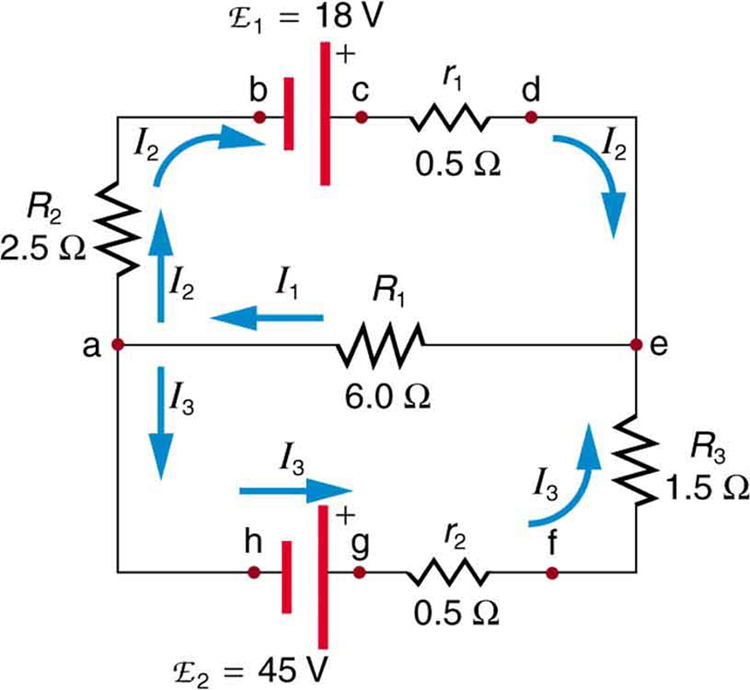# 21.3 Kirchhoff’s rules  (Page 3/8)

 Page 3 / 8

## Calculating current: using kirchhoff’s rules

Find the currents flowing in the circuit in [link] .This circuit is similar to that in [link] , but the resistances and emfs are specified. (Each emf is denoted by script E.) The currents in each branch are labeled and assumed to move in the directions shown. This example uses Kirchhoff’s rules to find the currents.

Strategy

This circuit is sufficiently complex that the currents cannot be found using Ohm’s law and the series-parallel techniques—it is necessary to use Kirchhoff’s rules. Currents have been labeled ${I}_{1}$ , ${I}_{2}$ , and ${I}_{3}$ in the figure and assumptions have been made about their directions. Locations on the diagram have been labeled with letters a through h. In the solution we will apply the junction and loop rules, seeking three independent equations to allow us to solve for the three unknown currents.

Solution

We begin by applying Kirchhoff’s first or junction rule at point a. This gives

${I}_{1}={I}_{2}+{I}_{3},$

since ${I}_{1}$ flows into the junction, while ${I}_{2}$ and ${I}_{3}$ flow out. Applying the junction rule at e produces exactly the same equation, so that no new information is obtained. This is a single equation with three unknowns—three independent equations are needed, and so the loop rule must be applied.

Now we consider the loop abcdea. Going from a to b, we traverse ${R}_{2}$ in the same (assumed) direction of the current ${I}_{2}$ , and so the change in potential is $-{I}_{2}{R}_{2}$ . Then going from b to c, we go from $–$ to +, so that the change in potential is $+{\text{emf}}_{1}$ . Traversing the internal resistance ${r}_{1}$ from c to d gives $-{I}_{2}{r}_{1}$ . Completing the loop by going from d to a again traverses a resistor in the same direction as its current, giving a change in potential of $-{I}_{1}{R}_{1}$ .

The loop rule states that the changes in potential sum to zero. Thus,

$-{I}_{2}{R}_{2}+{\text{emf}}_{1}-{I}_{2}{r}_{1}-{I}_{1}{R}_{1}=-{I}_{2}\left({R}_{2}+{r}_{1}\right)+{\text{emf}}_{1}-{I}_{1}{R}_{1}=0.$

Substituting values from the circuit diagram for the resistances and emf, and canceling the ampere unit gives

$-{3I}_{2}+\text{18}-{6I}_{1}=0.$

Now applying the loop rule to aefgha (we could have chosen abcdefgha as well) similarly gives

$+\phantom{\rule{0.25em}{0ex}}{I}_{1}{R}_{1}+{I}_{3}{R}_{3}+{I}_{3}{r}_{2}-{\text{emf}}_{2}\text{= +}{I}_{1}{R}_{1}+{I}_{3}\left({R}_{3}+{r}_{2}\right)-{\text{emf}}_{2}=0.$

Note that the signs are reversed compared with the other loop, because elements are traversed in the opposite direction. With values entered, this becomes

$+\phantom{\rule{0.25em}{0ex}}{6I}_{1}+{2I}_{3}-\text{45}=0.$

These three equations are sufficient to solve for the three unknown currents. First, solve the second equation for ${I}_{2}$ :

${I}_{2}=6-{2I}_{1}.$

Now solve the third equation for ${I}_{3}$ :

${I}_{3}=\text{22}\text{.}5-{3I}_{1}.$

Substituting these two new equations into the first one allows us to find a value for ${I}_{1}$ :

${I}_{1}={I}_{2}+{I}_{3}=\left(6-{2I}_{1}\right)+\left(\text{22}\text{.}5-{3I}_{1}\right)=\text{28}\text{.}5-{5I}_{1}.$

Combining terms gives

${6I}_{1}=\text{28}\text{.}5, and$
${I}_{1}=4\text{.}\text{75 A}.$

Substituting this value for ${I}_{1}$ back into the fourth equation gives

${I}_{2}=6-{2I}_{1}=6-9.50$
${I}_{2}=-3\text{.}\text{50 A}.$

The minus sign means ${I}_{2}$ flows in the direction opposite to that assumed in [link] .

Finally, substituting the value for ${I}_{1}$ into the fifth equation gives

${I}_{3}=\text{22.5}-{3I}_{1}=\text{22.5}-\text{14}\text{.}\text{25}$
${I}_{3}=8\text{.}\text{25 A}.$

Discussion

Just as a check, we note that indeed ${I}_{1}={I}_{2}+{I}_{3}$ . The results could also have been checked by entering all of the values into the equation for the abcdefgha loop.

What is the difference between a principle and a law
the law is universally proved. The principal depends on certain conditions.
Dr
what does the speedometer of a car measure ?
Car speedometer measures the rate of change of distance per unit time.
Moses
describe how a Michelson interferometer can be used to measure the index of refraction of a gas (including air)
using the law of reflection explain how powder takes the shine off a person's nose. what is the name of the optical effect?
WILLIAM
is higher resolution of microscope using red or blue light?.explain
WILLIAM
what is dimensional consistent
Mohammed
In engineering and science, dimensional analysis is the analysis of the relationships between different physical quantities by identifying their base quantities and units of measure and tracking these dimensions as calculations or comparisons are performed
syed
can sound wave in air be polarized?
Unlike transverse waves such as electromagnetic waves, longitudinal waves such as sound waves cannot be polarized. ... Since sound waves vibrate along their direction of propagation, they cannot be polarized
Astronomy
A proton moves at 7.50×107m/s perpendicular to a magnetic field. The field causes the proton to travel in a circular path of radius 0.800 m. What is the field strength?
derived dimenionsal formula
what is the difference between mass and weight
assume that a boy was born when his father was eighteen years.if the boy is thirteen years old now, how is his father in
Isru
what is airflow
derivative of first differential equation
why static friction is greater than Kinetic friction
draw magnetic field pattern for two wire carrying current in the same direction
An American traveler in New Zealand carries a transformer to convert New Zealand’s standard 240 V to 120 V so that she can use some small appliances on her trip.
What is the ratio of turns in the primary and secondary coils of her transformer?
nkombo
what is energy
Yusuf
How electric lines and equipotential surface are mutually perpendicular?
The potential difference between any two points on the surface is zero that implies È.Ŕ=0, Where R is the distance between two different points &E= Electric field intensity. From which we have cos þ =0, where þ is the angle between the directions of field and distance line, as E andR are zero. Thus
sorry..E and R are non zero...

#### Get Jobilize Job Search Mobile App in your pocket Now!ByByBy OpenStaxByBy OpenStaxByBy Richley CrapoBy Brooke DelaneyBy Melinda SalzerByBy John GabrieliBy Edgar Delgado